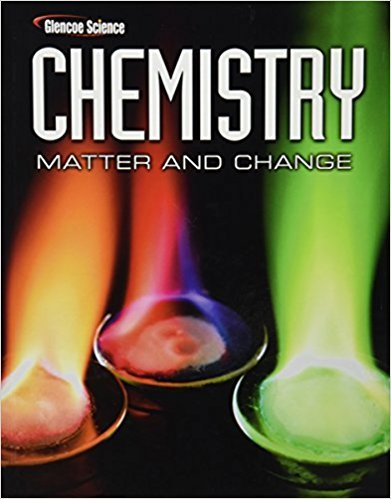×

×

# Solutions for Chapter 10: The Mole## Full solutions for Chemistry: Matter & Change | 1st Edition

ISBN: 9780078746376Solutions for Chapter 10: The Mole

Solutions for Chapter 10
4 5 0 284 Reviews
13
3
##### ISBN: 9780078746376

Since 216 problems in chapter 10: The Mole have been answered, more than 256362 students have viewed full step-by-step solutions from this chapter. Chapter 10: The Mole includes 216 full step-by-step solutions. Chemistry: Matter & Change was written by and is associated to the ISBN: 9780078746376. This textbook survival guide was created for the textbook: Chemistry: Matter & Change, edition: 1. This expansive textbook survival guide covers the following chapters and their solutions.

Key Chemistry Terms and definitions covered in this textbook
• actinide series.

Elements that have incompletely filled 5f subshells or readily give rise to cations that have incompletely filled 5f subshells. (7.9)

• boat conformation

A conformation of cyclohexane in which all bond angles are fairly close to 109.5° and many hydrogen atoms are eclipsing each other.

• chemical equilibrium

A state of dynamic balance in which the rate of formation of the products of a reaction from the reactants equals the rate of formation of the reactants from the products; at equilibrium the concentrations of the reactants and products remain constant. (Section 4.1;Chapter 15: Introduction)

• chemical reactions

Processes in which one or more substances are converted into other substances; also called chemical changes. (Section 1.3)

• chiral

An object that is not superimposable on its mirror image.

• Clemmensen reduction

A reaction in which a carbonyl group is completely reduced and replaced with two hydrogen atoms.

• coordination compound

A compound containing a metal ion bonded to a group of surrounding molecules or ions that act as ligands. (Section 23.2)

• coordination number.

In a crystal lattice it is defined as the number of atoms (or ions) surrounding an atom (or ion) (11.4). In coordination compounds it is defined as the number of donor atoms surrounding the central metal atom in a complex. (23.3)

• critical pressure

The pressure at which a gas at its critical temperature is converted to a liquid state. (Section 11.4)

• crystal-field theory

A theory that accounts for the colors and the magnetic and other properties of transition-metal complexes in terms of the splitting of the energies of metal ion d orbitals by the electrostatic interaction with the ligands. (Section 23.6)

• dynamic equilibrium

A state of balance in which opposing processes occur at the same rate. (Section 11.5)

• hydrolysis

A reaction with water. When a cation or anion reacts with water, it changes the pH. (Sections 16.9 and 24.4)

• joule (J)

The SI unit of energy, 1 kg@m2 >s2. A related unit is the calorie: 4.184 J = 1 cal. (Section 5.1)

• limiting reactant (limiting reagent)

The reactant present in the smallest stoichiometric quantity in a mixture of reactants; the amount of product that can form is limited by the complete consumption of the limiting reactant. (Section 3.7)

• N-Terminal amino acid

The amino acid at the end of a polypeptide chain having the free !NH2 group

• node

Points in an atom at which the electron density is zero. For example, the node in a 2s orbital is a spherical surface. (Section 6.6)

• Nucleotide

A nucleoside in which a molecule of phosphoric acid is esterifi ed with an !OH of the monosaccharide, most commonly either the 39!OH or the 59!OH.

• oxidation number (oxidation state)

A positive or negative whole number assigned to an element in a molecule or ion on the basis of a set of formal rules; to some degree it reflects the positive or negative character of that atom. (Section 4.4)

• redox (oxidation–reduction) reaction

A reaction in which certain atoms undergo changes in oxidation states. The substance increasing in oxidation state is oxidized; the substance decreasing in oxidation state is reduced. (Section 4.4; Chapter 20: Introduction)

• Ribosomal RNA (rRNA)

A ribonucleic acid found in ribosomes, the sites of protein synthesi